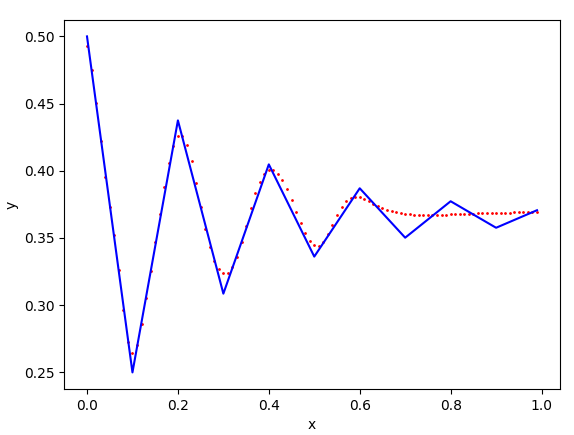# Neural Architecture Search with NNIIn this post, we will show how to perform hyper-parameter search using an automated machine learning (AutoML) tool — NNI (for Neural Network Intelligence) open-sourced by Microsoft.

Neural architecture search (NAS) is a difficult challenge in deep learning. Many of us have experienced that for a given dataset, a network may initially struggle to learn. But with a simple change of a hyper-parameter, the learning can become very effective. Manually tweaking hyper-parameters including architecture is time-consuming and challenging even though to some it can also be a lot of fun. Recently, automatic hyper-parameter tuning has become more and more popular, as it provides an efficient mechanism to solve NAS at scale.

In this post, we will show how to perform hyper-parameter search using an automated machine learning (AutoML) tool — NNI (for Neural Network Intelligence) open-sourced by Microsoft. I just got started to play with NNI and I have liked it so far. Here I want to share how I use NNI to search for optimal hyper-parameters and architectures.

We will build a neural network to approximate math functions, as an example. Neural networks with one or more hidden layers are known as function approximators for continuous functions , assuming certain non-linearity conditions of activation functions are met. Shallow networks such as the ones with a single hidden layer are not as efficient as width-bound deep networks (for example ). Michael Nielsen has given a nice, accessible explanation of how neural nets can approximate functions.

## Simple Nonlinear Function

We begin with a simple nonlinear target function y = x². We use a fully connected network with a few hidden layers to learn this function within the range [0, 1].

Below is code to implement this function as a PyTorch dataset. Input data and ground-truth labels are represented by tensors of shape (1,1), where the two components are channels and data dimension, respectively. They are both 1 since x and y hold only scalar values. If you have experience in image-based neural networks, you can think of the data as a single-channel, single-pixel image.

## All About Artificial Neural Networks – What, Why, and How?

Artificial Neural Network is a learning system that seeks to emulate the human brain. Here is an overview of ANN, critical to the discipline of Artificial Intelligence.

## Activation Functions in Neural Network

A concise analysis on roles and types of activation functions in neural networks. Detailed pros and cons of 5 popularly used ones in Artificial Neural Network.

## Introduction to Artificial Neural Networks for Beginners

Introduction to Artificial Neural Networks for Beginners. Understanding the concepts of Neural Networks.

## Introduction to Neural Networks

There has been hype about artificial intelligence, machine learning, and neural networks for quite a while now. This will not be a math-heavy introduction because I just want to build the idea here.

## Types Of Activation Functions used in Neural Network?

Discuss various types of activation functions and what are the types of problems one might encounter while using each of them.